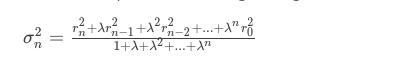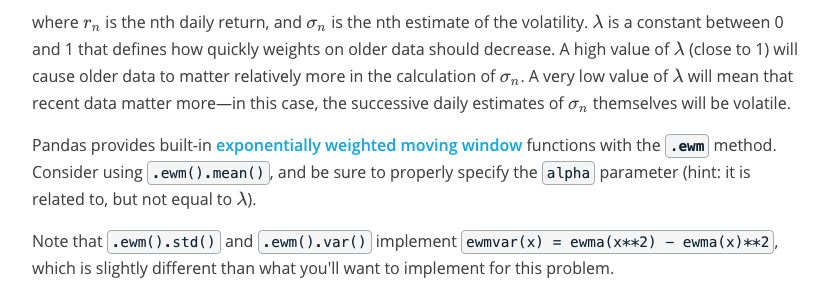# AI For Trading: Quize:Estimate Volatility (27)

## Estimate Volatility

Create an exponential moving average model of volatility. Use the following formula:import pandas as pd
import numpy as np

def estimate_volatility(prices, l):
"""Create an exponential moving average model of the volatility of a stock
price, and return the most recent (last) volatility estimate.

Parameters
----------
prices : pandas.Series
A series of adjusted closing prices for a stock.

l : float
The 'lambda' parameter of the exponential moving average model. Making
this value smaller will cause the model to weight older terms less
relative to more recent terms.

Returns
-------
last_vol : float
The last element of your exponential moving averge volatility model series.

"""
# TODO: Implement the exponential moving average volatility model and return the last value.

pass

def test_run(filename='data.csv'):
"""Test run get_most_volatile() with stock prices from a file."""
prices = pd.read_csv(filename, parse_dates=['date'], index_col='date', squeeze=True)
print("Most recent volatility estimate: {:.6f}".format(estimate_volatility(prices, 0.7)))

if __name__ == '__main__':
test_run()